# Voltage and Current

Next Page: Meters

Also See: Multimeters | Ohm's Law

Voltage and Current are vital to understanding electronics, but they are quite hard to grasp because we cannot see them directly.

## Voltage is the Cause, Current is the Effect

Voltage attempts to make a current flow, and current will flow if the circuit is complete. Voltage is sometimes described as the 'push' or 'force' of the electricity, it isn't really a force but this may help you to imagine what is happening. It is possible to have voltage without current, but current cannot flow without voltage.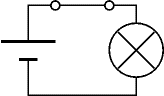Voltage and Current
The switch is closed making
a complete circuit so
current can flow.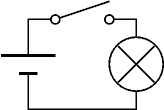Voltage but No Current
The switch is open so
the circuit is broken and
current cannot flow.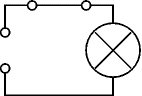No Voltage and No Current
Without the cell there is
no source of voltage so
current cannot flow.

## Voltage, V

• Voltage is a measure of the energy carried by the charge.
Strictly: voltage is the 'energy per unit charge'.
• The proper name for voltage is potential difference or p.d. for short, but this term is rarely used in electronics.
• Voltage is supplied by the battery (or power supply).
• Voltage is used up in components, but not in wires.
• We say voltage across a component.
• Voltage is measured in volts, V.
• Voltage is measured with a voltmeter, connected in parallel.
• The symbol V is used for voltage in equations.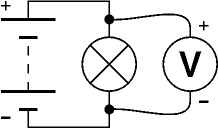Connecting a voltmeter in parallel

### Voltage at a point and 0V (zero volts)

Voltage is a difference between two points, but in electronics we often refer to voltage at a point meaning the voltage difference between that point and a reference point of 0V (zero volts).

Zero volts could be any point in the circuit, but to be consistent it is normally the negative terminal of the battery or power supply. You will often see circuit diagrams labelled with 0V as a reminder.

You may find it helpful to think of voltage like height in geography. The reference point of zero height is the mean (average) sea level and all heights are measured from that point. The zero volts in an electronic circuit is like the mean sea level in geography.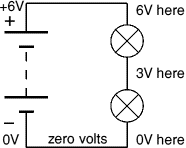#### Zero volts for circuits with a dual supply

Some circuits require a dual supply with three supply connections as shown in the diagram. For these circuits the zero volts reference point is the middle terminal between the two parts of the supply.

On complex circuit diagrams using a dual supply the earth symbol is often used to indicate a connection to 0V, this helps to reduce the number of wires drawn on the diagram.

The diagram shows a ±9V dual supply, the middle terminal is 0V.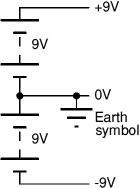## Current, I

• Current is the rate of flow of charge.
• Current is not used up, what flows into a component must flow out.
• We say current through a component.
• Current is measured in amps (amperes), A.
• Current is measured with an ammeter, connected in series.
To connect in series you must break the circuit and put the ammeter acoss the gap, as shown in the diagram.
• The symbol I is used for current in equations.
Why is the letter I used for current? ... please see FAQ.

1A (1 amp) is quite a large current for electronics, so mA (milliamp) is often used. m (milli) means 'thousandth':

1mA = 0.001A, or 1000mA = 1A

The need to break the circuit to connect in series means that ammeters are difficult to use on soldered circuits. Most testing in electronics is done with voltmeters which can be easily connected without disturbing circuits.Connecting an ammeter in series

## Voltage and Current for components in Series

• Voltages add up for components connected in series.
• Currents are the same through all components connected in series.

In this circuit the 4V across the resistor and the 2V across the LED add up to the battery voltage: 2V + 4V = 6V.

The current through all parts (battery, resistor and LED) is 20mA.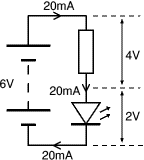## Voltage and Current for components in Parallel

• Voltages are the same across all components connected in parallel.
• Currents add up for components connected in parallel.

In this circuit the battery, resistor and lamp all have 6V across them.

The 30mA current through the resistor and the 60mA current through the lamp add up to the 90mA current through the battery.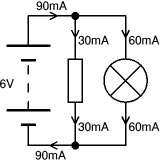Next Page: Meters | Study